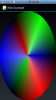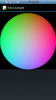# Android QuestionWheel Color Generate

#### Star-Dust

##### Expert
Longtime User
Hello everyone,
I tried to write a code that allows me to draw the attached image on a canvas. But with disappointing results, does anyone know how to give me a hand?or•dost

#### eurojam

##### Well-Known Member
Longtime User
make a quiz of that question, it will be interesting to see the different solutions....best regards from toscana under olive trees...
stefan

•LucaMs, DonManfred and Star-Dust

#### Star-Dust

##### Expert
Longtime User
make a quiz of that question, it will be interesting to see the different solutions....best regards from toscana under olive trees...
stefan
Question too hard

#### Erel

##### B4X founder
Staff member
Longtime User
(You shouldn't use polls for technical questions.)Simple color picker written in 10 lines of code:
B4X:
``````Sub Activity_Create(FirstTime As Boolean)
cvsColors.Initialize(pnlColors)
cvsOverlay.Initialize(pnlOverlay)
End Sub

Sub pnlColors_Touch (Action As Int, X As Float, Y As Float)
Dim cx = pnlColors.Width / 2, cy = pnlColors.Height / 2 As Float
If Sqrt(Power(x - cx, 2) + Power(y - cy, 2)) > pnlColors.Width / 2 Then Return
Dim clr As Int = cvsColors.Bitmap.GetPixel(X, Y)
Activity.Color = clr
cvsOverlay.DrawColor(Colors.Transparent)
cvsOverlay.DrawCircle(X, Y, 20dip, Colors.Black, False, 3dip)
pnlOverlay.Invalidate
End Sub``````

You haven't posted why you need the color wheel so I assumed that you want to create a color picker.

#### Attachments

• ColorPicker.zip
397.9 KB · Views: 1,288

#### Star-Dust

##### Expert
Longtime User
I just saw the source code just because I was out of the office. I have to upload the image already ready, I thought there was an algorithm that generated the image.
But if I create a library and add pictures to the resource, then do I find myself in the app where I use the library?

Longtime User
Last edited:

#### LucaMs

##### Expert
Longtime User
Thank you LucaMS, but using Google Translate you do not understand anything. Is it a yes or no?
E allora scrivi nel forum italiano, no?
La domanda è se puoi inserire in una libreria dei file di risorse, in questo caso l'immagine usata per il ColorPicker? La risposta è sì, ed il link che ti ho fornito spiega come fare.

Then write in the italian forumIf you need help I can help you for only 200€ / hour(create a new thread in the italian forum, if you need help).

Longtime User

#### Star-Dust

##### Expert
Longtime User
code is Java

B4X:
``````Public Bitmap GetImage() {
BitmapWrapper bw = new BitmapWrapper();
bw.Initialize2(this.getClass().getResourceAsStream("droid.png"));

Return bw.getObject();
}``````

Longtime User

#### Star-Dust

##### Expert
Longtime User
After hours of work, with this code:
B4X:
``````Private Sub pattern As Bitmap
Dim Btm As Bitmap
Dim Can As Canvas
Dim Width As Int =100dip
Dim Height As Int =100dip
Dim Raggio As Int = 50dip
Dim X, Y As Int
Dim C1, C2, C3 As Int

Btm.InitializeMutable(Width,Height)
Can.Initialize2(Btm)

For An=0 To 255
For rag=0 To 255
X=Raggio+Cos((2*cPI/3)*(An/255))*(Raggio*rag/255)
y=Raggio+Sin((2*cPI/3)*(An/255))*(Raggio*rag/255)
C1=(255-rag)*(An/255)
C2=(255-rag)*((255-An)/255)
C3=255-Abs(An-127.5)*2
Can.DrawPoint(x,y,Colors.RGB(C3,C1,C2))
Next
Next

For An=0 To 255
For rag=0 To 255
X=Raggio+Cos((2*cPI/3)+(2*cPI/3)*(An/255))*(Raggio*rag/255)
y=Raggio+Sin((2*cPI/3)+(2*cPI/3)*(An/255))*(Raggio*rag/255)
C1=(255-rag)*(An/255)
C2=(255-rag)*((255-An)/255)
C3=255-Abs(An-127.5)*2
Can.DrawPoint(x,y,Colors.RGB(C1,C2,C3))
Next
Next

For An=0 To 255
For rag=0 To 255
X=Raggio+Cos((4*cPI/3)+(2*cPI/3)*(An/255))*(Raggio*rag/255)
y=Raggio+Sin((4*cPI/3)+(2*cPI/3)*(An/255))*(Raggio*rag/255)
C1=(255-rag)*(An/255)
C2=(255-rag)*((255-An)/255)
C3=255-Abs(An-127.5)*2
Can.DrawPoint(x,y,Colors.RGB(C2,C3,C1))
Next
Next

Return Can.Bitmap
End Sub``````

I get this picture. That's not what I wanted exactly. Maybe someone has a better idea#### Star-Dust

##### Expert
Longtime User
Try new code:

B4X:
``````Private Sub pattern As Bitmap
Dim Btm As Bitmap
Dim Can As Canvas
Dim Width As Int = 200dip
Dim Height As Int = 200dip
'Center Point
Dim CenterX As Int = Width/2
Dim CenterY As Int = Height/2
Dim radius As Int = CenterX * CenterY
' RED
Dim redX As Int = Width
Dim redY As Int = Height /2
Dim redRad As Int = Width*Width
' Green
Dim greenX As Int = 0
Dim greenY As Int = Height/2
Dim greenRad As Int = Width*Width
'Blue
Dim blueX As Int = Width/ 2
Dim blueY As Int = Height
Dim blueRad As Int = Width*Width

Btm.InitializeMutable(Width,Height)
Can.Initialize2(Btm)

For i=0 To Width-1
For j=0 To Height-1
Dim a As Int = i - CenterX
Dim b As Int = j - CenterY

Dim distance As Int = a * a + b * b
Dim distance As Int = a * a + b * b
Dim rdx As Int = i - redX
Dim rdy As Int = j - redY
Dim redDist As Int = (rdx * rdx + rdy * rdy)
Dim redVal As Int = (255 - ((redDist / redRad) * 256))

Dim gdx As Int = i - greenX
Dim gdy As Int = j - greenY
Dim greenDist As Int = (gdx * gdx + gdy * gdy)
Dim greenVal As Int = (255 - ((greenDist / greenRad) * 256))

Dim bdx As Int = i - blueX
Dim bdy As Int = j - blueY
Dim blueDist As Int = (bdx * bdx + bdy * bdy)
Dim blueVal As Int = (255 - ((blueDist / blueRad) * 256))

Dim c As Int =  Colors.rgb(redVal, greenVal, blueVal)

'float hsbVals[] = Color.RGBtoHSB(c.getRed(), c.getGreen(), c.getBlue(), Null);
'Color highlight = Color.getHSBColor(hsbVals, hsbVals, 1);
'img.setRGB(i, j, RGBtoHEX(highlight));
Can.DrawPoint(i,j,c)
Else
Can.DrawPoint(i,j,Colors.RGB(0,0,0))
End If
Next
Next

Return Can.Bitmap
End Sub``````•DavideV

#### LucaMs

##### Expert
Longtime User
"Chi va con lo zoppo, impara a zoppicare" (an Italian saying)
"Whoever goes with lame learns to limp"

You lose time and consume your neurons when Erel has already resolved it; there is no problem including the bitmap in your library, so just use his code.

#### Star-Dust

##### Expert
Longtime User
In the meantime it has become a personal issue between me and programming. There is an algorithm that can produce a similar graph. Why do not I have to be able to find it? In the end I'm a math ... but very basically ... in some ocean maybe ... in some abyss ... 20 thousand leagues under the seas.

In any case it is not clear from the link you gave me to add a resource to a library.

Last edited:

Longtime User

#### Star-Dust

##### Expert
Longtime User
I find a new solutionI think this solution is best

B4X:
``````Dim Panel As Panel

Panel.Initialize("")
Panel.SetBackgroundImage(ColorWheel)

SUB
B4X:
``````Sub ColorWheel As Bitmap
Dim Can As Canvas
Dim Bt As Bitmap
Dim D As Int = 240
Dim Center As Int = D/4
Dim Radius As Int = D/4
Dim X,Y,X2,Y2 As Int

Bt.InitializeMutable(d/2,d/2)
Can.Initialize2(Bt)

For h=0 To d
For s=0 To d-10

'Can.DrawPoint(x,y ,HSLtoRGB2(H,s,100,255))
Can.DrawLine(X,Y,X2,Y2,HSLtoRGB(H,s+10,100,255),3)
Next
Next
Return Can.Bitmap
End Sub

Sub HSLtoRGB(Hue As Int, Saturation As Int, Luminance As Int, Alpha As Int ) As Int
Dim temp3(3) As Double , Red As Int, Green As Int, Blue As Int ,temp1 As Double, temp2 As Double ,n As Int
Dim pHue As Double, pSat As Double, pLum As Double , pRed As Double, pGreen As Double, pBlue As Double

pHue = Min(239, Hue) / 239
pSat = Min(239, Saturation) / 239
pLum = Min(239, Luminance) / 239

If pSat = 0 Then
pRed = pLum
pGreen = pLum
pBlue = pLum
Else
If pLum < 0.5 Then
temp2 = pLum * (1 + pSat)
Else
temp2 = pLum + pSat - pLum * pSat
End If
temp1 = 2 * pLum - temp2

temp3(0) = pHue + 1 / 3
temp3(1) = pHue
temp3(2) = pHue - 1 / 3

For n = 0 To 2
If temp3(n) < 0 Then temp3(n) = temp3(n) + 1
If temp3(n) > 1 Then temp3(n) = temp3(n) - 1

If 6 * temp3(n) < 1 Then
temp3(n) = temp1 + (temp2 - temp1) * 6 * temp3(n)
Else
If 2 * temp3(n) < 1 Then
temp3(n) = temp2
Else
If 3 * temp3(n) < 2 Then
temp3(n) = temp1 + (temp2 - temp1) * ((2 / 3) - temp3(n)) * 6
Else
temp3(n) = temp1
End If
End If
End If
Next

pRed = temp3(0)
pGreen = temp3(1)
pBlue = temp3(2)
End If

Red = pRed * 255
Green = pGreen * 255
Blue = pBlue * 255

Return Colors.ARGB(Alpha, Red,Green,Blue)
End Sub``````Last edited:
•Solutions Development and LucaMs